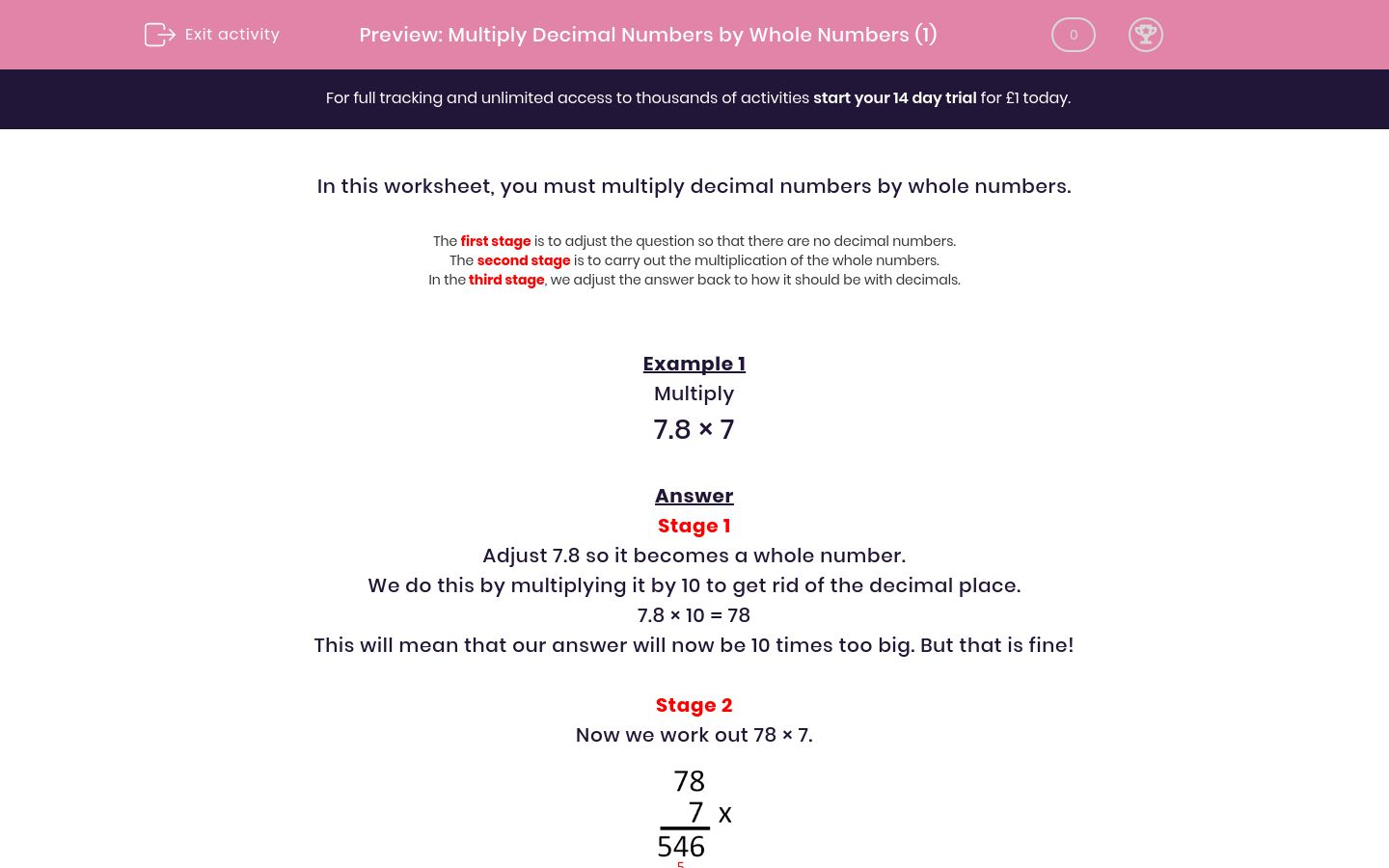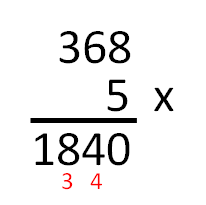# Multiply Decimal Numbers by Whole Numbers (1)

In this worksheet, students multiply one-digit numbers with up to two decimal places by whole numbersKey stage:  KS 2

Curriculum topic:   Maths and Numerical Reasoning

Curriculum subtopic:   Decimals

Difficulty level:#### Worksheet Overview

In this worksheet, you must multiply decimal numbers by whole numbers.

• The first stage is to adjust the question so that there are no decimal numbers.
• The second stage is to carry out the multiplication of the whole numbers.
• In the third stage, we adjust the answer back to how it should be with decimals.

Example 1

Multiply

7.8 × 7

Answer

Stage 1

Adjust 7.8 so it becomes a whole number.

We do this by multiplying it by 10 to get rid of the decimal place.

7.8 × 10 = 78

This will mean that our answer will now be 10 times too big. But that is fine!

Stage 2

Now we work out 78 × 7.Stage 3

Now, remember that this is 10 times too big.

So we divide 546 ÷ 10 = 54.6.

7.8 × 7 = 54.6

Example 2

Multiply

3.68 × 5

Answer

Stage 1

Adjust 3.68 so it becomes a whole number.

We do this by multiplying it by 100 to get rid of the 2 decimal places.

3.68 × 100 = 368

This will mean that our answer will now be 100 times too big. But that is fine!

Stage 2

Now we work out 368 × 5.Stage 3

Now, remember that this is 100 times too big.

So we divide 1840 ÷ 100 = 18.40 = 18.4.

3.68 × 5 = 18.4

### What is EdPlace?

We're your National Curriculum aligned online education content provider helping each child succeed in English, maths and science from year 1 to GCSE. With an EdPlace account you’ll be able to track and measure progress, helping each child achieve their best. We build confidence and attainment by personalising each child’s learning at a level that suits them.

Get startedTry an activity or get started for free